• Call Now

1800-102-2727•

# Contact Force, Normal Force, Normal Force In Different Cases, Practice Problems, FAQs

How do you measure your weight? Obviously using a weighing machine. Do you know the mechanism of the weighing machine? Actually the weighing machine measures nothing but the normal force acting on it. So when you stand on the machine the normal force is applied by you on the machine which is nothing but your weight. While solving the problems in mechanics we deal with normal forces a lot. Let’s understand this force in detail.

• Contact force
• Normal force
• Calculation of normal force in different cases
• Practice problems
• FAQs

## Contact Force

Contact force is a force that is applied by objects in contact with each other. The contact force acts on a point of direct contact between the two objects. Contact force is governed by Newton’s Laws. Contact force is responsible for most of the interactions we experience in daily life.

• This force occurs due to the interaction of particles of the body with each other.
• This force is electromagnetic in nature.
• These forces act in random directions which can be resolved in two components along the surface and are normal to it.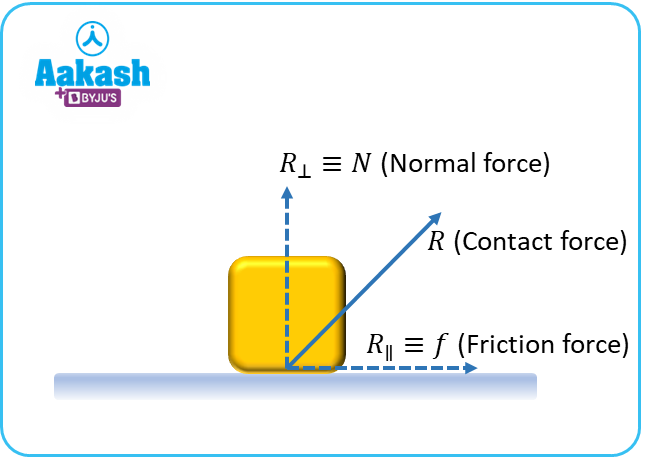Components of Contact Force:

1. Forces parallel to the surface are called Friction forces.
2. Force perpendicular to the surface is called Normal Force.

## Normal Force

The normal force or normal reaction is the support force exerted upon an object that is in contact with another fixed or stable object. That’s why this force is also called the Support Force. This force is a perpendicular component of contact force.

Example: Object kept on horizontal plane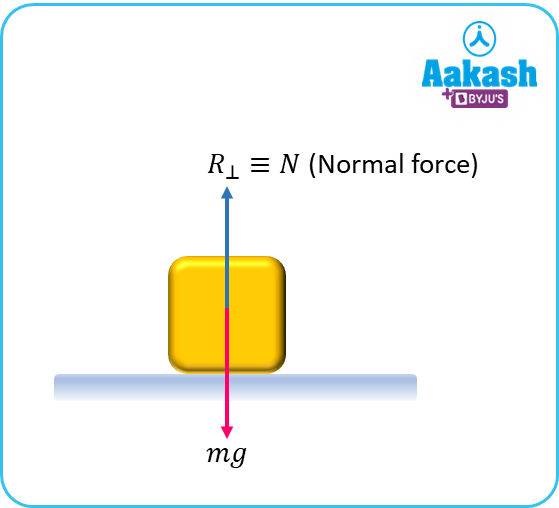Here normal force applied by plank on the object is perpendicular to the surface. This force is balanced by the weight of an object. So in this case, N=mg

Note:

• Normal reactions are always pushing in nature.
• It is always normal (90 degrees) to the surface.

## Calculation of Normal force in Different Cases

Case1: Object kept on inclined plane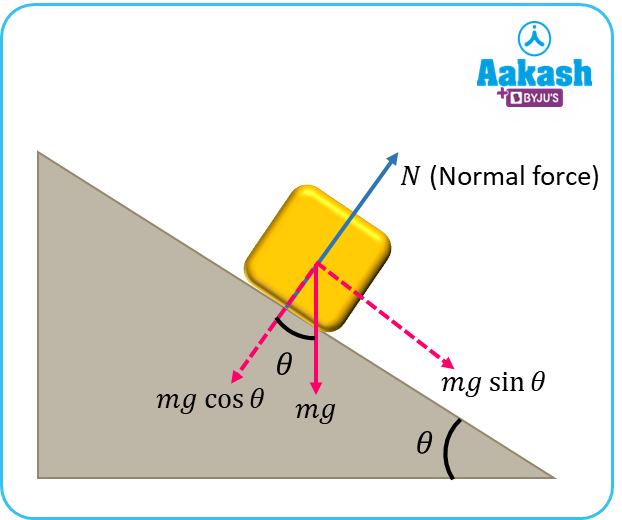Here also normal force applied by the wedge on the object is perpendicular to the surface. This force is balanced by the component of weight in that direction. So in this case, N=mg cos

Case2: Block over block system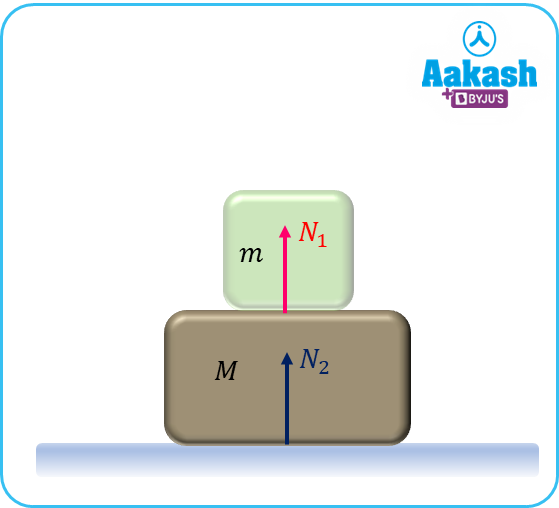Normal force by M on the m will be equal to the weight of m.

So N1=mg

Normal force by the ground on the M will be equal to the combined weight of M and m.

So ${N}_{2}=\left(M+m\right)g$

## Practice problems

Question.1 Friction force and contact force on the block is 9 N and 15 N. Then find the normal force on the block?

We know the relation,

Question.2 A contact force of 100 N is acting on the block at an angle of 37o with respect to horizontal. Find the normal force on the block?

Normal force is the perpendicular component of contact force so,

Question.3 Find the normal force by the ground on the lowest block in the given diagram?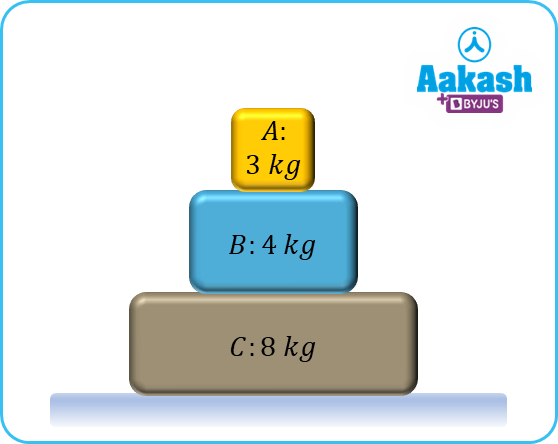Lower Block:

To balance the forces in a vertical direction, the normal force on the block C will be equal to the weight of A, B and C.

So,

Question.4 Find the normal force applied by the wedge on the blocks as shown in the figure?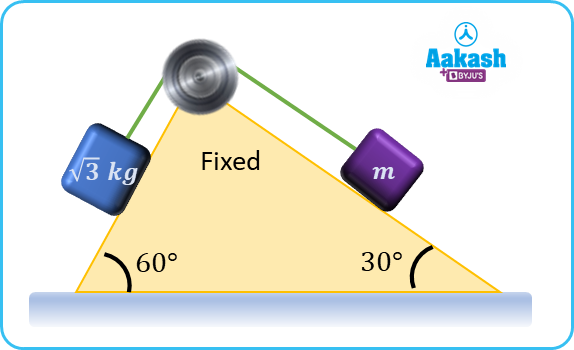For 3 Kg Block

For m Block

## FAQs

Question.1 What is contact force?
Contact force is a force that is applied by objects in contact with each other. The contact force acts on a point of direct contact between the two objects.

Question.2 What is the direction of normal force?
Normal reactions are always pushing in nature and its direction is always perpendicular to the surface.

Question.3 Is normal force always equal to the weight of an object?
No, depending upon the orientation of objects and the given system, normal force may differ from weight of an object.

Question.4 In what case the normal force on the object will be zero?
If an object is kept in such a way that it is not in contact with any other surface or object then normal force on it will be zero.Talk to our expert
Resend OTP Timer =
By submitting up, I agree to receive all the Whatsapp communication on my registered number and Aakash terms and conditions and privacy policy